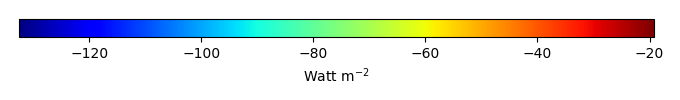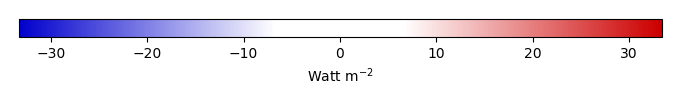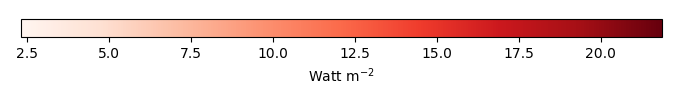# Mean State

Period Mean (original grids) [Watt m-2]
Model Period Mean (intersection) [Watt m-2]
Model Period Mean (complement) [Watt m-2]
Benchmark Period Mean (intersection) [Watt m-2]
Benchmark Period Mean (complement) [Watt m-2]
Bias [Watt m-2]
RMSE [Watt m-2]
Phase Shift [months]
Bias Score 
RMSE Score 
Seasonal Cycle Score 
Spatial Distribution Score 
Interannual Variability Score 
Overall Score 
Benchmark [-] -56.8
CLM5PHSOFF [-] -66.4 -66.5 -57.0 -52.3 -9.72 16.8 1.70 0.453 0.451 0.753 0.980 0.840 0.655
CLM5PHSON [-] -66.5 -66.5 -57.0 -52.3 -9.56 16.5 1.67 0.441 0.458 0.762 0.975 0.822 0.652
Period Mean (original grids) [Watt m-2]
Model Period Mean (intersection) [Watt m-2]
Model Period Mean (complement) [Watt m-2]
Benchmark Period Mean (intersection) [Watt m-2]
Benchmark Period Mean (complement) [Watt m-2]
Bias [Watt m-2]
RMSE [Watt m-2]
Phase Shift [months]
Bias Score 
RMSE Score 
Seasonal Cycle Score 
Spatial Distribution Score 
Interannual Variability Score 
Overall Score 
Benchmark [-] -87.0
CLM5PHSOFF [-] -97.6 -98.2 -87.4 -58.4 -10.6 21.8 0.276 0.668 0.606 0.974 0.736 0.752 0.724
CLM5PHSON [-] -97.3 -98.0 -87.4 -58.4 -10.2 22.1 0.309 0.676 0.590 0.971 0.811 0.720 0.726
Period Mean (original grids) [Watt m-2]
Model Period Mean (intersection) [Watt m-2]
Model Period Mean (complement) [Watt m-2]
Benchmark Period Mean (intersection) [Watt m-2]
Benchmark Period Mean (complement) [Watt m-2]
Bias [Watt m-2]
RMSE [Watt m-2]
Phase Shift [months]
Bias Score 
RMSE Score 
Seasonal Cycle Score 
Spatial Distribution Score 
Interannual Variability Score 
Overall Score 
Benchmark [-] -81.4
CLM5PHSOFF [-] -92.2 -92.5 -82.3 -62.6 -10.2 20.4 0.732 0.571 0.527 0.913 0.968 0.734 0.707
CLM5PHSON [-] -91.9 -92.0 -82.3 -62.6 -7.58 19.7 0.810 0.617 0.506 0.901 0.972 0.712 0.702
Period Mean (original grids) [Watt m-2]
Model Period Mean (intersection) [Watt m-2]
Model Period Mean (complement) [Watt m-2]
Benchmark Period Mean (intersection) [Watt m-2]
Benchmark Period Mean (complement) [Watt m-2]
Bias [Watt m-2]
RMSE [Watt m-2]
Phase Shift [months]
Bias Score 
RMSE Score 
Seasonal Cycle Score 
Spatial Distribution Score 
Interannual Variability Score 
Overall Score 
Benchmark [-] -37.2
CLM5PHSOFF [-] -40.3 -40.3 -36.9 -41.0 -3.45 16.2 1.16 0.708 0.414 0.850 0.964 0.882 0.705
CLM5PHSON [-] -39.5 -39.6 -36.9 -41.0 -3.38 16.5 1.11 0.715 0.401 0.859 0.967 0.882 0.704
Period Mean (original grids) [Watt m-2]
Model Period Mean (intersection) [Watt m-2]
Model Period Mean (complement) [Watt m-2]
Benchmark Period Mean (intersection) [Watt m-2]
Benchmark Period Mean (complement) [Watt m-2]
Bias [Watt m-2]
RMSE [Watt m-2]
Phase Shift [months]
Bias Score 
RMSE Score 
Seasonal Cycle Score 
Spatial Distribution Score 
Interannual Variability Score 
Overall Score 
Benchmark [-] -44.6
CLM5PHSOFF [-] -48.1 -48.2 -43.2 -49.2 -5.03 10.2 1.87 0.357 0.342 0.716 0.968 0.782 0.585
CLM5PHSON [-] -49.7 -49.7 -43.2 -49.2 -5.81 10.4 1.88 0.350 0.344 0.716 0.981 0.778 0.585
Period Mean (original grids) [Watt m-2]
Model Period Mean (intersection) [Watt m-2]
Model Period Mean (complement) [Watt m-2]
Benchmark Period Mean (intersection) [Watt m-2]
Benchmark Period Mean (complement) [Watt m-2]
Bias [Watt m-2]
RMSE [Watt m-2]
Phase Shift [months]
Bias Score 
RMSE Score 
Seasonal Cycle Score 
Spatial Distribution Score 
Interannual Variability Score 
Overall Score 
Benchmark [-] -42.1
CLM5PHSOFF [-] -53.4 -53.4 -41.9 -51.7 -11.4 16.2 0.732 0.495 0.507 0.923 0.998 0.893 0.721
CLM5PHSON [-] -52.1 -52.1 -41.9 -51.7 -10.1 15.7 0.716 0.538 0.487 0.918 0.994 0.893 0.720
Period Mean (original grids) [Watt m-2]
Model Period Mean (intersection) [Watt m-2]
Model Period Mean (complement) [Watt m-2]
Benchmark Period Mean (intersection) [Watt m-2]
Benchmark Period Mean (complement) [Watt m-2]
Bias [Watt m-2]
RMSE [Watt m-2]
Phase Shift [months]
Bias Score 
RMSE Score 
Seasonal Cycle Score 
Spatial Distribution Score 
Interannual Variability Score 
Overall Score 
Benchmark [-] -67.1
CLM5PHSOFF [-] -73.2 -73.0 -67.3 -59.4 -5.77 16.6 0.982 0.643 0.505 0.861 0.987 0.804 0.718
CLM5PHSON [-] -72.9 -72.6 -67.3 -59.4 -4.47 16.3 1.02 0.677 0.496 0.853 0.992 0.807 0.721
Period Mean (original grids) [Watt m-2]
Model Period Mean (intersection) [Watt m-2]
Model Period Mean (complement) [Watt m-2]
Benchmark Period Mean (intersection) [Watt m-2]
Benchmark Period Mean (complement) [Watt m-2]
Bias [Watt m-2]
RMSE [Watt m-2]
Phase Shift [months]
Bias Score 
RMSE Score 
Seasonal Cycle Score 
Spatial Distribution Score 
Interannual Variability Score 
Overall Score 
Benchmark [-] -71.2
CLM5PHSOFF [-] -79.3 -79.8 -72.6 -53.4 -7.30 18.8 0.828 0.639 0.558 0.893 0.960 0.722 0.722
CLM5PHSON [-] -80.3 -80.5 -72.6 -53.4 -5.12 18.3 0.861 0.679 0.546 0.890 0.955 0.710 0.721
Period Mean (original grids) [Watt m-2]
Model Period Mean (intersection) [Watt m-2]
Model Period Mean (complement) [Watt m-2]
Benchmark Period Mean (intersection) [Watt m-2]
Benchmark Period Mean (complement) [Watt m-2]
Bias [Watt m-2]
RMSE [Watt m-2]
Phase Shift [months]
Bias Score 
RMSE Score 
Seasonal Cycle Score 
Spatial Distribution Score 
Interannual Variability Score 
Overall Score 
Benchmark [-] -31.1
CLM5PHSOFF [-] -39.3 -39.4 -31.1 -32.1 -8.25 16.1 0.832 0.595 0.439 0.919 0.948 0.896 0.706
CLM5PHSON [-] -38.9 -39.0 -31.1 -32.1 -7.87 16.1 0.817 0.608 0.431 0.919 0.961 0.898 0.708
Period Mean (original grids) [Watt m-2]
Model Period Mean (intersection) [Watt m-2]
Model Period Mean (complement) [Watt m-2]
Benchmark Period Mean (intersection) [Watt m-2]
Benchmark Period Mean (complement) [Watt m-2]
Bias [Watt m-2]
RMSE [Watt m-2]
Phase Shift [months]
Bias Score 
RMSE Score 
Seasonal Cycle Score 
Spatial Distribution Score 
Interannual Variability Score 
Overall Score 
Benchmark [-] -96.8
CLM5PHSOFF [-] -97.1 -97.3 -99.4 -63.9 2.03 15.8 0.495 0.671 0.546 0.927 0.950 0.756 0.733
CLM5PHSON [-] -99.6 -99.6 -99.4 -63.9 3.14 15.8 0.545 0.685 0.540 0.920 0.963 0.745 0.732
Period Mean (original grids) [Watt m-2]
Model Period Mean (intersection) [Watt m-2]
Model Period Mean (complement) [Watt m-2]
Benchmark Period Mean (intersection) [Watt m-2]
Benchmark Period Mean (complement) [Watt m-2]
Bias [Watt m-2]
RMSE [Watt m-2]
Phase Shift [months]
Bias Score 
RMSE Score 
Seasonal Cycle Score 
Spatial Distribution Score 
Interannual Variability Score 
Overall Score 
Benchmark [-] -55.6
CLM5PHSOFF [-] -60.4 -60.3 -55.1 -60.8 -5.21 11.9 0.722 0.612 0.478 0.913 0.989 0.866 0.723
CLM5PHSON [-] -58.2 -58.2 -55.1 -60.8 -3.61 11.6 0.745 0.654 0.470 0.909 0.973 0.869 0.724
Period Mean (original grids) [Watt m-2]
Model Period Mean (intersection) [Watt m-2]
Model Period Mean (complement) [Watt m-2]
Benchmark Period Mean (intersection) [Watt m-2]
Benchmark Period Mean (complement) [Watt m-2]
Bias [Watt m-2]
RMSE [Watt m-2]
Phase Shift [months]
Bias Score 
RMSE Score 
Seasonal Cycle Score 
Spatial Distribution Score 
Interannual Variability Score 
Overall Score 
Benchmark [-] -52.6
CLM5PHSOFF [-] -70.9 -70.9 -65.2 -46.5 -5.75 17.6 0.968 0.555 0.497 0.866 0.949 0.776 0.690
CLM5PHSON [-] -71.2 -71.2 -65.2 -46.5 -4.70 17.3 0.974 0.577 0.491 0.865 0.944 0.769 0.689
Period Mean (original grids) [Watt m-2]
Model Period Mean (intersection) [Watt m-2]
Model Period Mean (complement) [Watt m-2]
Benchmark Period Mean (intersection) [Watt m-2]
Benchmark Period Mean (complement) [Watt m-2]
Bias [Watt m-2]
RMSE [Watt m-2]
Phase Shift [months]
Bias Score 
RMSE Score 
Seasonal Cycle Score 
Spatial Distribution Score 
Interannual Variability Score 
Overall Score 
Benchmark [-] -121.
CLM5PHSOFF [-] -107. -107. -122. -81.3 14.8 25.7 0.671 0.565 0.529 0.911 0.784 0.634 0.659
CLM5PHSON [-] -108. -108. -122. -81.3 15.4 25.9 0.675 0.562 0.529 0.911 0.819 0.635 0.664
Period Mean (original grids) [Watt m-2]
Model Period Mean (intersection) [Watt m-2]
Model Period Mean (complement) [Watt m-2]
Benchmark Period Mean (intersection) [Watt m-2]
Benchmark Period Mean (complement) [Watt m-2]
Bias [Watt m-2]
RMSE [Watt m-2]
Phase Shift [months]
Bias Score 
RMSE Score 
Seasonal Cycle Score 
Spatial Distribution Score 
Interannual Variability Score 
Overall Score 
Benchmark [-] -34.3
CLM5PHSOFF [-] -42.9 -42.9 -34.2 -36.5 -8.74 15.3 0.805 0.573 0.460 0.897 0.830 0.882 0.683
CLM5PHSON [-] -42.1 -42.1 -34.2 -36.5 -8.40 15.3 0.806 0.584 0.451 0.895 0.841 0.881 0.684
Period Mean (original grids) [Watt m-2]
Model Period Mean (intersection) [Watt m-2]
Model Period Mean (complement) [Watt m-2]
Benchmark Period Mean (intersection) [Watt m-2]
Benchmark Period Mean (complement) [Watt m-2]
Bias [Watt m-2]
RMSE [Watt m-2]
Phase Shift [months]
Bias Score 
RMSE Score 
Seasonal Cycle Score 
Spatial Distribution Score 
Interannual Variability Score 
Overall Score 
Benchmark [-] -70.7
CLM5PHSOFF [-] -82.6 -82.8 -73.4 -57.5 -9.52 16.8 1.09 0.503 0.480 0.831 0.995 0.805 0.682
CLM5PHSON [-] -84.6 -84.6 -73.4 -57.5 -7.82 16.0 1.09 0.531 0.481 0.828 0.996 0.796 0.685
Period Mean (original grids) [Watt m-2]
Model Period Mean (intersection) [Watt m-2]
Model Period Mean (complement) [Watt m-2]
Benchmark Period Mean (intersection) [Watt m-2]
Benchmark Period Mean (complement) [Watt m-2]
Bias [Watt m-2]
RMSE [Watt m-2]
Phase Shift [months]
Bias Score 
RMSE Score 
Seasonal Cycle Score 
Spatial Distribution Score 
Interannual Variability Score 
Overall Score 
Benchmark [-] -53.2
CLM5PHSOFF [-] -64.2 -64.1 -52.9 -58.5 -11.2 14.7 0.750 0.409 0.485 0.878 0.994 0.891 0.690
CLM5PHSON [-] -61.8 -61.7 -52.9 -58.5 -8.67 13.0 0.738 0.496 0.471 0.881 0.989 0.891 0.700
Period Mean (original grids) [Watt m-2]
Model Period Mean (intersection) [Watt m-2]
Model Period Mean (complement) [Watt m-2]
Benchmark Period Mean (intersection) [Watt m-2]
Benchmark Period Mean (complement) [Watt m-2]
Bias [Watt m-2]
RMSE [Watt m-2]
Phase Shift [months]
Bias Score 
RMSE Score 
Seasonal Cycle Score 
Spatial Distribution Score 
Interannual Variability Score 
Overall Score 
Benchmark [-] -42.5
CLM5PHSOFF [-] -54.2 -54.2 -42.4 -45.4 -11.8 16.2 0.542 0.504 0.526 0.938 0.994 0.866 0.726
CLM5PHSON [-] -52.8 -52.8 -42.4 -45.4 -10.2 15.3 0.536 0.551 0.511 0.939 0.981 0.861 0.726
Period Mean (original grids) [Watt m-2]
Model Period Mean (intersection) [Watt m-2]
Model Period Mean (complement) [Watt m-2]
Benchmark Period Mean (intersection) [Watt m-2]
Benchmark Period Mean (complement) [Watt m-2]
Bias [Watt m-2]
RMSE [Watt m-2]
Phase Shift [months]
Bias Score 
RMSE Score 
Seasonal Cycle Score 
Spatial Distribution Score 
Interannual Variability Score 
Overall Score 
Benchmark [-] -80.3
CLM5PHSOFF [-] -88.1 -87.9 -80.8 -54.9 -7.23 18.1 0.220 0.695 0.576 0.976 0.934 0.768 0.754
CLM5PHSON [-] -86.8 -86.6 -80.8 -54.9 -5.44 18.1 0.247 0.720 0.566 0.971 0.940 0.771 0.756
Period Mean (original grids) [Watt m-2]
Model Period Mean (intersection) [Watt m-2]
Model Period Mean (complement) [Watt m-2]
Benchmark Period Mean (intersection) [Watt m-2]
Benchmark Period Mean (complement) [Watt m-2]
Bias [Watt m-2]
RMSE [Watt m-2]
Phase Shift [months]
Bias Score 
RMSE Score 
Seasonal Cycle Score 
Spatial Distribution Score 
Interannual Variability Score 
Overall Score 
Benchmark [-] -43.7
CLM5PHSOFF [-] -52.5 -52.4 -43.6 -47.7 -8.76 13.9 1.67 0.469 0.451 0.766 0.768 0.772 0.613
CLM5PHSON [-] -51.8 -51.7 -43.6 -47.7 -8.13 13.1 1.56 0.463 0.460 0.786 0.873 0.753 0.633
Period Mean (original grids) [Watt m-2]
Model Period Mean (intersection) [Watt m-2]
Model Period Mean (complement) [Watt m-2]
Benchmark Period Mean (intersection) [Watt m-2]
Benchmark Period Mean (complement) [Watt m-2]
Bias [Watt m-2]
RMSE [Watt m-2]
Phase Shift [months]
Bias Score 
RMSE Score 
Seasonal Cycle Score 
Spatial Distribution Score 
Interannual Variability Score 
Overall Score 
Benchmark [-] -64.5
CLM5PHSOFF [-] -73.7 -74.1 -64.8 -56.3 -9.33 15.8 0.826 0.580 0.477 0.892 0.903 0.840 0.695
CLM5PHSON [-] -72.2 -72.6 -64.8 -56.3 -7.17 14.8 0.801 0.647 0.476 0.897 0.900 0.815 0.702
Period Mean (original grids) [Watt m-2]
Model Period Mean (intersection) [Watt m-2]
Model Period Mean (complement) [Watt m-2]
Benchmark Period Mean (intersection) [Watt m-2]
Benchmark Period Mean (complement) [Watt m-2]
Bias [Watt m-2]
RMSE [Watt m-2]
Phase Shift [months]
Bias Score 
RMSE Score 
Seasonal Cycle Score 
Spatial Distribution Score 
Interannual Variability Score 
Overall Score 
Benchmark [-] -80.0
CLM5PHSOFF [-] -85.0 -84.6 -83.0 -54.0 -1.47 20.5 0.775 0.502 0.440 0.887 0.905 0.769 0.657
CLM5PHSON [-] -85.7 -85.3 -83.0 -54.0 0.359 19.8 0.785 0.544 0.442 0.886 0.892 0.773 0.663

# Temporally integrated period mean

BENCHMARK MEANMODEL MEANBIASBIAS SCORERMSERMSE SCOREBENCHMARK INTERANNUAL VARIABILITYMODEL INTERANNUAL VARIABILITYINTERANNUAL VARIABILITY SCOREBENCHMARK MAX MONTHMODEL MAX MONTHDIFFERENCE IN MAX MONTHSEASONAL CYCLE SCORESPATIAL TAYLOR DIAGRAMMODEL COLORS# Spatially integrated regional mean

MODEL COLORSREGIONAL MEANANNUAL CYCLEMONTHLY ANOMALYANNUAL CYCLE# All Models

BenchmarkCLM5PHSOFFCLM5PHSON# Data Information

creation_date: Tue Jul 1 08:25:00 PDT 2014

source_file: This product is generated from monthly 1 degree GEWEX SRB Radiation observations